NEET  >  Test: Average Velocity & Average Speed

# Test: Average Velocity & Average Speed

Test Description

## 5 Questions MCQ Test Physics Class 11 | Test: Average Velocity & Average Speed

Test: Average Velocity & Average Speed for NEET 2023 is part of Physics Class 11 preparation. The Test: Average Velocity & Average Speed questions and answers have been prepared according to the NEET exam syllabus.The Test: Average Velocity & Average Speed MCQs are made for NEET 2023 Exam. Find important definitions, questions, notes, meanings, examples, exercises, MCQs and online tests for Test: Average Velocity & Average Speed below.
Solutions of Test: Average Velocity & Average Speed questions in English are available as part of our Physics Class 11 for NEET & Test: Average Velocity & Average Speed solutions in Hindi for Physics Class 11 course. Download more important topics, notes, lectures and mock test series for NEET Exam by signing up for free. Attempt Test: Average Velocity & Average Speed | 5 questions in 5 minutes | Mock test for NEET preparation | Free important questions MCQ to study Physics Class 11 for NEET Exam | Download free PDF with solutions
 1 Crore+ students have signed up on EduRev. Have you?
Test: Average Velocity & Average Speed - Question 1

### Which of the following statements are incorrect? (i) Average velocity is path length divided by time interval. (ii) In general, speed is greater than the magnitude of the velocity. (iii) A particle moving in a given direction with a non-zero velocity can have zero speed. (iv) The magnitude of average velocity is the average speed.

Detailed Solution for Test: Average Velocity & Average Speed - Question 1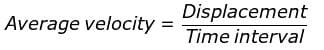A particle moving in a given direction with non-zero velocity cannot have zero speed. In general, average speed is not equal to magnitude of average velocity. However it can be so if the motion is along a straight line without change in direction.

Test: Average Velocity & Average Speed - Question 2

### A car is moving along a straight line OP as shown in the figure. It moves from O to P in 18 s and returns from P to Q in 6 s. Which of the following statements is not correct regarding the motion of the car?Detailed Solution for Test: Average Velocity & Average Speed - Question 2From O to P and back to Q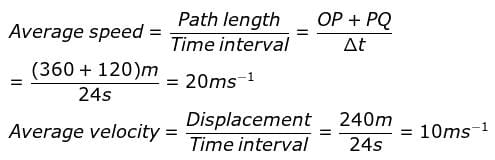From O to P and come back to O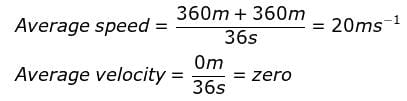Test: Average Velocity & Average Speed - Question 3

### The area under velocity-time graph for a particle in a given interval of time represents

Detailed Solution for Test: Average Velocity & Average Speed - Question 3

The area under the velocity-time graph represents the displacement over a given time interval.

Test: Average Velocity & Average Speed - Question 4

A cyclist moving on a circular track of radius 40m completes half a revolution in 40s. Its average velocity is

Detailed Solution for Test: Average Velocity & Average Speed - Question 4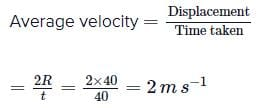Test: Average Velocity & Average Speed - Question 5

The position of an object moving along x-axis is given by x = a + bt2 , where a = 8.5 m and b = 2.5 m s−2 and t is measured in seconds. The average velocity of the object between t = 2 s and t = 4 s is

Detailed Solution for Test: Average Velocity & Average Speed - Question 5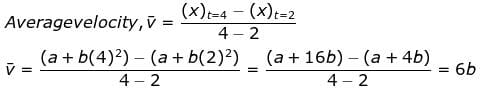6(2.5)m s-1 = 15m s-1

## Physics Class 11

127 videos|464 docs|210 tests
 Use Code STAYHOME200 and get INR 200 additional OFF Use Coupon Code
Information about Test: Average Velocity & Average Speed Page
In this test you can find the Exam questions for Test: Average Velocity & Average Speed solved & explained in the simplest way possible. Besides giving Questions and answers for Test: Average Velocity & Average Speed, EduRev gives you an ample number of Online tests for practice

## Physics Class 11

127 videos|464 docs|210 tests

### How to Prepare for NEET

Read our guide to prepare for NEET which is created by Toppers & the best Teachers